##### Quantum Physics For Dummies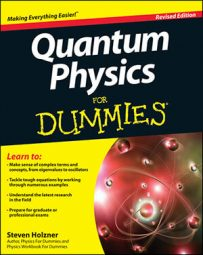In quantum physics, if you know the boundary conditions of a square well, you can find theenergy levels of an electron.

The equation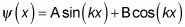tells you that you have to use the boundary conditions to find the constants A and B. What are the boundary conditions? The wave function must disappear at the boundaries of an infinite square well, so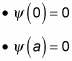The fact that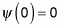tells you right away that B must be zero because cos(0) = 1. And the fact that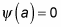tells you that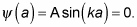Because sine is zero when its argument is a multiple of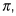this means that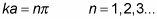Note that although n = 0 is technically a solution, it yields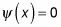for all x, which is not normalizable, so it’s not a physical solution — the physical solutions begin with n = 1.

This equation can also be written as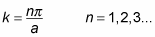And because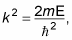you have the following equation, where n = 1, 2, 3, ... — those are the allowed energy states. These are quantized states, corresponding to the quantum numbers 1, 2, 3, and so on: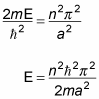Note that the first physical state corresponds to n = 1, which gives you this next equation: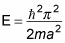This is the lowest physical state that the particles can occupy. Just for kicks, put some numbers into this, assuming that you have an electron, mass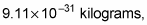confined to an infinite square well of width of the order of the Bohr radius (the average radius of an electron’s orbit in a hydrogen atom); let’s say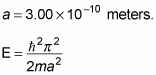gives you this energy for the ground state: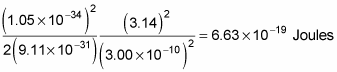That’s a very small amount, about 4 electron volts (eV — the amount of energy one electron gains falling through 1 volt). Even so, it’s already on the order of the energy of the ground state of an electron in the ground state of a hydrogen atom (13.6 eV), so you can say you’re certainly in the right quantum physics ballpark now.